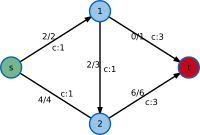# Network Flow

A very common problem in graphs and networks is the computation of flows. Examples include the flow of goods in a logistics system, information in a communication network, or natural gas in a pipeline network. Usually, one is interested either in computing a maximal flow, where the amount of goods to be transported along an edge is bounded by a capacity limit and one wants to route the maximal flow possible from some source node to a given target. Alternatively, one might be interested in routing a given amount of flow in a cost-efficient way, that problem is known as minimum cost flow.##### Cycle-Cancelling Algorithm

The Cycle-Cancelling Algorithm yields a flow of minimal cost.##### Ford-Fulkerson Algorithm

The Ford-Fulkerson Algorithm computes the maximal flow in a network.